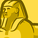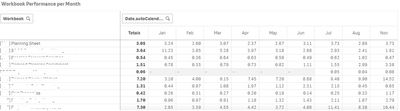# App Development

Announcements
April 22, 2PM EST: Learn about GeoOperations in Qlik Sense SaaS READ MORE
cancel
Showing results for
Did you mean:Contributor III

## Trend expression for monthly measure

Hi all,

I don't know much about QSense expressions language and I need the expression to get the trend (positive or negative) of a monthly measure.

The data is the following (records of what users are consulting and the response time)

Table: Query Detail

 Date User Workbook Elapsed Time May Mark Planning Sheet 2.4 May Lily Planning Sheet 2.3 June john Planning Sheet 2.1 July Lily Planning Sheet 3 August Mark Planning Sheet 4 August Lily Planning Sheet 5

With this table, I would get that the Planning Sheet Workbook has the following Average Elapsed Time per month:

 Date Average Elapsed Time May 2.35 June 2.1 July 3 August 4.5

So, I want to build an expression that tells me if the tendence is increasing or decreasing. In this case is increasing.

I don't know if I need something like the first month-last month and see if there's a difference, or also taking into account the weight of usage...

Thanks!!!

Labels (7)

• ### Tutorial

1 Solution

Accepted SolutionsMVP

I am answering for difference between first and last month

first create two fields like below in calendar

month(Date) as Month,
num(month(Date)) as MonthNum,

then create calculated dimension

=aggr(if((sum({<MonthNum={"\$(=max(MonthNum))"}>}Sales)-sum({<MonthNum={"\$(=min(MonthNum))"}>}Sales))>0,'Positive','Negative'),Text)

5 RepliesMVP

What exactly you want to achieve? Can you please elaborate and provide exact output you need?Contributor III
Author

I now have a pivot table (See the table attached)

In this table I have the average elapsed time of the different Workbooks for every month. (Dimensions Workbook and Month, Measure Average Elapsed Time). The data is gathered from the example Query Detail table I posted.

I would like to get the following:

Adding a column in this table with only two outputs available for each Workbook:

1- POSITIVE: When the trend over the month is decreasing, negative

2- NEGATIVE: When the trend over the month is increasing, positiveMVP

How would you decide increasing or decreasing? Comparing current month with previous month?  If current month Avg is more than previous month Avg then increasing?Contributor III
Author

Comparing the Average Elapsed Time between the first month (january) and the actual,

Or, the one would be best:

Comparing the same but with the weight of Count(workbook) to give more importance to the months that have been more used.

ThanksMVP

I am answering for difference between first and last month

first create two fields like below in calendar

month(Date) as Month,
num(month(Date)) as MonthNum,

then create calculated dimension

=aggr(if((sum({<MonthNum={"\$(=max(MonthNum))"}>}Sales)-sum({<MonthNum={"\$(=min(MonthNum))"}>}Sales))>0,'Positive','Negative'),Text)Tags
Community Browser